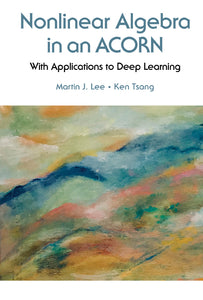# Nonlinear Algebra In An Acorn: With Applications To Deep Learning

Martin J Lee

Format: Print Book

ISBN: 9789813271517

• SGD 75.97
Unit price per
Tax included.

A simple algorithm for solving a set of nonlinear equations by matrix algebra has been discovered recently — first by transforming them into an equivalent matrix equation and then finding the solution analytically in terms of the inverse matrix of this equation. With this newly developed ACORN (Adaptive Constrained Optimal Robust Nonlinear) algorithm, it is possible to minimize the objective function [constructed from the functions in the nonlinear set of equations] without computing its derivatives.This book will present the details of ACORN algorithm and how it is used to solve large scale nonlinear equations with an innovative approach ACORN Magic [minimization algorithms gathered in a cloud].The ultimate motivation of this work is its application to optimization. In recent years, with the advances in big-data, optimization becomes an even more powerful tool in knowledge discovery. ACORN Magic is the perfect choice in this kind of application because of that fact that it is fast, robust and simple enough to be embedded in any type of machine learning program.

Format: Hardcover
No of Pages: 92
Imprint: World Scientific
Publication date: 20180905